## General Question# What is the percentage of 170 out of 440?

Asked by silky1 (1510) May 17th, 2012

I need to know what percentage rate this would be so I can pay this guy the correct amount.

Observing members: 0Composing members: 0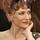Divide 170 by 440; or 17 by 44, which is the same thing.

Jeruba (52019)“Great Answer” (4) Flag as…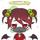(100/440)*170

ragingloli (49248)“Great Answer” (3) Flag as…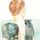Just a tip: You can type “170/440=” or any other simple math problem into Google and it will automatically tell you the answer.

To find the percent, you just divide 170 into 440 and multiply by 100. (170/440)(100)=38.63%

Fly (8726)“Great Answer” (5) Flag as…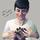Here’s my foolproof method for percentage problems, or how to translate English to math.

Another way to phrase your question is “What percent of 440 is 170?”

Each word in that sentence converts directly to a math symbol:
What: this is what you’re looking to find, call it x.
Percent: “per” means division, “cent” means 100; percent literally just means divide by 100.
Of: protip, “of” translates to mutiplication in most cases.
Is: i.e. equals, this is your equal sign.

So “What percent of 440 is 170?” translates to:

X/100 * 440 = 170
Then solve for x by dividing by 440 and multiplying by 100.

Works every time.

Mariah (25876)“Great Answer” (5) Flag as…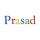= (170/440)*100 percent

prasad (3859)“Great Answer” (0) Flag as…or